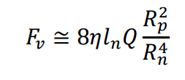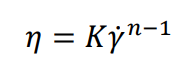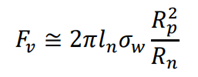# May the Force Be With You, Injection Force That is!

May 4, 2021

Rheology as a tool can contribute to formulation characterization as well as formulation analysis and application development. Injection force estimates are one such rheological tool. One of the most common uses of viscosity is to assess the ability to deliver pharmaceutical formulations to the patient by injection. However, a key limitation for antibody therapeutics is the high concentrations necessary to achieve an effective dose. High concentrations of protein lead to large increases in viscosity, which can make injection more difficult. Depending on the device used for injection, the cutoff viscosity of the formulation can be between 20 and 40 cP (mPa-s). A significant component of formulation research and development is rheology modification to ensure that therapeutics can be delivered without an excessive injection force. (RheoSense, Injectability Foundation)

Incorporating injectability analysis earlier in therapeutic development can guide development and mitigate the risk of developing a formulation that is impossible to deliver. The first step in incorporating injectability analysis is to first measure the formulations’ viscosity over a wide range of shear rates to determine the fluid’s behavior. The second step is determining the shear rates that correspond to the injection flow rate and needle gauge. Once those components are known then you will be able to estimate the injection force. However, the first question you may ask is “I don’t know where to begin to estimate injectability?” Injectability and viscosity are closely related. So, if you know the viscosity of your sample as well as the rate of injection and syringe geometry, then you will be able to estimate the injection force.

The simplest injection force estimation case is one for shear independent fluids, also known as Newtonian fluids. Injection force estimates only calculate the viscous or hydrodynamic contribution to the injection force. This estimation does not include the friction component of the injection force, because there is too much variability between syringes, needles, and lubricants for us to accurately estimate the frictional component of injectability. The injection force estimation equation for Newtonian Fluids is:If your fluid is non-Newtonian, then the first step to determining injection force is to make sure that your non-Newtonian data has been corrected. If your viscometer or rheometer induces an inhomogeneous flow on your fluid, then you must perform a correction. The appropriate correction for flow through a rectangular channel (as used in RheoSense VROC instruments) is the Weissenberg-Rabinowitsch-Mooney (WRM) correction, which is an analysis method that can determine the “true shear rate,” using the “apparent shear rate” measured by the viscometer. (Macosko, 1994)

A very common deviation from Newtonian behavior is the power-law fluid. The power law fluid model is used to fit non-Newtonian data across shear rates where there is no evidence of a Newtonian plateau region. Fitting to the power law model is appropriate where the measured data is entirely within the shear-thinning regime across all the shear rates tested. The power law equation is:Shear rate dependent viscosity data of complex fluids often follows a form more complicated than the power-law. For example, a viscosity profile can include both a shear thinning region as well as a full or partial plateau. Since pharmaceutical formulations typically fall under the classification of complex fluids, this is an import situation to address. The general injection force estimation equation for non-Newtonian Fluids is:With viscosity data you can apply the right equations to calculate the viscous contribution to the injection force for Newtonian, power-law, and general non-Newtonian fluids. Calculating an accurate injection force estimation will help to ensure that suitable therapeutic candidates are not discarded but continue to move forward in the development process.

Want to learn more about testing your samples’ viscosity to calculate injection force? Download our application note describing a protocol on testing your samples’ viscosity over a wide range of shear rates that are relevant to estimating injectability with the VROC® initium one plus!Written by: Dr. Zachary Imam, RheoSense Research Scientist

## You May Also Like

These Stories on Viscosity Measurements